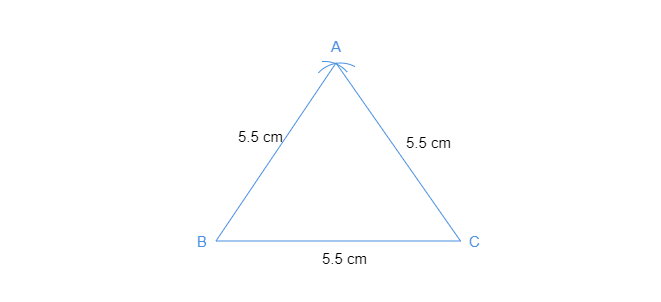# Construct an equilateral triangle of side $5.5\ cm$.

Given: An equilateral triangle of side $5.5\ cm$.

To do: To construct an equilateral triangle of side $5.5\ cm$.

Steps of construction:

• Draw a line segment $BC$ of length $5.5\ cm$.
• Cut an arc of radius $5\ cm$ from point $B$ and an arc of $5.5\ cm$ from point $C$.
• Name the point of intersection of arcs as point $A$.• Now, join $AC$ and $BC$.

$\Delta ABC$ is the required triangle.